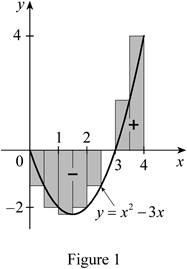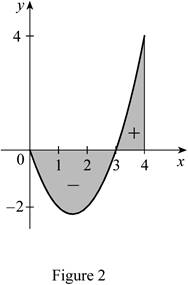# an approximation to the integral ∫ 0 4 ( x 2 − 3 x ) d x using a Riemann sum with right endpoints.### Single Variable Calculus: Concepts...

4th Edition
James Stewart
Publisher: Cengage Learning
ISBN: 9781337687805### Single Variable Calculus: Concepts...

4th Edition
James Stewart
Publisher: Cengage Learning
ISBN: 9781337687805

#### Solutions

Chapter 5.2, Problem 26E

a)

To determine

## To find: an approximation to the integral ∫04(x2−3x)dx using a Riemann sum with right endpoints.

Expert Solution

The approximation to the integral 04(x23x)dx using a Riemann sum with right endpoints is 1.5_.

### Explanation of Solution

Given:

The integral function is, 04(x23x)dx.

The number of sub intervals as n=8.

The upper limit is 4 and lower limit is 0.

Theorem 4: If f is integrable on [a, b], then

abf(x)dx=limni=1nf(xi)Δx

Where Δx=ban and xi=a+iΔx

Calculation:

The integral function is,

04(x23x)dx

The Riemann sum with right endpointsformula is,

abf(x)dx=i=1nf(xi*)Δx (1)

Calculate the subinterval (Δx) using the formula:

Δx=ban

Here, b is upper limit, a is lower limit, and n is number of subintervals.

Substitute 4 for b, 0 for a, and 8 for n.

Δx=408=0.5

Hence, the subinterval length is 0.5 for the limits [0,4].

The subinterval values are 0.5, 1.0, 1.5, 2.0, 2.5,3.0, 3.5,and 4.0for x1, x2, x3, x4, x5, x6, x7, and x8

Calculate the approximation to the integral using a Riemann sum with right endpoints:

Substitute 0.5 for Δx and 8 for n in Equation (1).

abf(x)dx=i=18[f(xi)]0.5=[f(x1)+f(x2)+f(x3)+f(x4)+f(x5)+f(x6)+f(x7)+f(x8)]0.5 (2)

Apply the subinterval values in Equation (3).

abf(x)dx=[f(0.5)+f(1.0)+f(1.5)+f(2.0)+f(2.5)+f(3.0)+f(3.5)+f(4.0)]0.5 (3)

Consider f(x)=x23x (4)

Calculate f(0.5)

Substitute 0.5 for x in Equation (4).

f(0.5)=(0.5)23(0.5)=0.25

Calculate f(1.0):

Substitute 1.0 for x in Equation (4).

f(1.0)=(1.0)23(1.0)=2.00

Calculate f(1.5)

Substitute 1.5 for x in Equation (4).

f(1.5)=(1.5)23(1.5)=2.25

Calculate f(2.0):

Substitute 2.0 for x in Equation (4).

f(2.0)=(2.0)23(2.0)=2.00

Calculate f(2.5)

Substitute 2.5 for x in Equation (4).

f(2.5)=(2.5)23(2.5)=1.25

Calculate f(3.0):

Substitute 3.0 for x in Equation (4).

f(3.0)=(3.0)23(3.0)=0.00

Calculate f(3.5)

Substitute 3.5 for x in Equation (4).

f(3.5)=(3.5)23(3.5)=1.75

Calculate f(4.0):

Substitute 4.0 for x in Equation (4).

f(4.0)=(4.0)23(4.0)=4.00

Substitute -1.25, -2.00, -2.25, -2.00, -1.25, 0.00, 1.75, and 4.00 for f(0.5),f(1.0),f(1.5), f(2.0), f(2.5), f(3.0), f(3.5), and f(4.0) respectively in Equation (3).

abf(x)dx=[1.252.002.252.001.25+0.00+1.75+4.00]0.5=1.5

Hence, the approximation to the integral 04(x23x)dx using a Riemann sum with right endpoints is 1.5_.

b)

To determine

### To draw: a diagram similar to figure 3 to illustrate the approximation in part (a).

Expert Solution

A diagram similar to figure 3 to illustrate the approximation in part (a) is drawn.

### Explanation of Solution

Calculation:

Show the equation as below:

y=x23x (5)

Plot a graph for the equation y=x23x using the calculation as follows:

Calculate y value using Equation (5).

Substitute 0 for x in Equation (5).

y=(0)23(0)=0

Hence, the co-ordinate of (x,y) is (0,0).

Calculate y value using Equation (5).

Substitute 1 for x in Equation (5).

y=(1)23(1)=2

Hence, the co-ordinate of (x,y) is (1,2).

Calculate y value using Equation (5).

Substitute 2 for x in Equation (5).

y=(2)23(2)=2

Hence, the co-ordinate of (x,y) is (2,2).

Calculate y value using Equation (5).

Substitute 3 for x in Equation (5).

y=(3)23(3)=0

Hence, the co-ordinate of (x,y) is (3,0).

Calculate y value using Equation (5).

Substitute 4 for x in Equation (5).

y=(4)23(4)=4

Hence, the co-ordinate of (x,y) is (4,4).

Draw the region as shown in Figure 1.c)

To determine

### To evaluate: the integral ∫04(x2−3x)dx using Theorem 4.

Expert Solution

The evaluation of the integral 04(x23x)dx using Theorem 4 is 83_.

### Explanation of Solution

Calculation:

The Theorem 4 is,

abf(x)dx=limni=1nf(xi)Δx (6)

Calculate the subinterval (Δx) using the formula:

Δx=ban (7)

Here, b is upper limit, a is lower limit, and n is number of subintervals.

Substitute 4 for b, and 0 for a.

Δx=40n=4n

Calculate the xi using the formula:

xi=a+iΔx (8)

Substitute 4n for Δx and 0 for a in Equation (7)

xi=0+i4n=4in

The integral function is,

04(x23x)dx (9)

Apply the Theorem 4 in Equation (5).

04(x23x)dx=limni=1n(xi23xi)Δx (10)

Substitute 4in for xi and 4n for Δx in Equation (10).

04(x23x)dx=limni=1n[(4in)23(4in)](4n)=limn4n[16n2i=1ni212ni=1ni]=limn[64n3×n(n+1)(2n+1)648n2×n(n+1)2]=limn[323(1+1n)(2+1n)24(1+1n)]

=(323×2)24=83

Hence, the evaluation of the integral 04(x23x)dx using Theorem 4 is 83_.

d)

To determine

### To draw: a diagram similar to figure 4 to illustrate the integral in part (c) as a difference of areas.

Expert Solution

A diagram similar to figure 4 to illustrate the integral in part (c) as a difference of areas is drawn.

### Explanation of Solution

Calculation:

Draw the region as shown in Figure 2.Refer Figure 2

Positive sign (+) refers area A1 and negative sign () refers area A2.

The integral function is as follows:

04(x23x)dx=A1A2.

### Have a homework question?

Subscribe to bartleby learn! Ask subject matter experts 30 homework questions each month. Plus, you’ll have access to millions of step-by-step textbook answers!